Скачать презентацию Chapter 6 International Arbitrage and Interest rate Parity

76556a8d5c527158b72768bfe95528af.ppt

• Количество слайдов: 29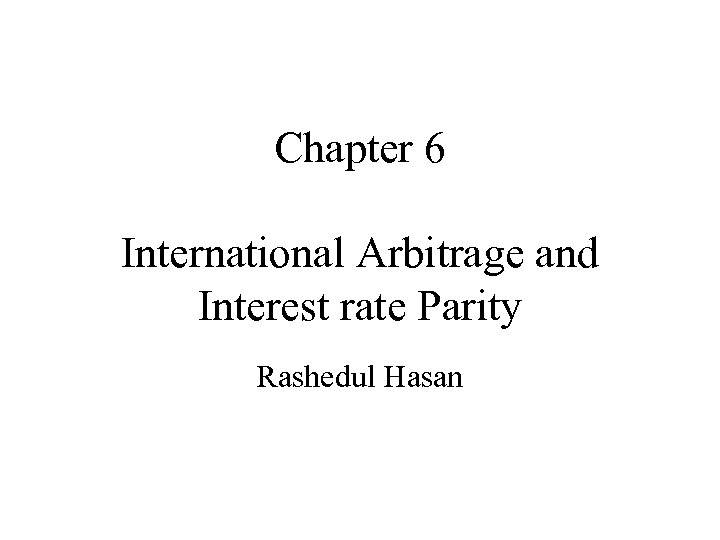Chapter 6 International Arbitrage and Interest rate Parity Rashedul Hasan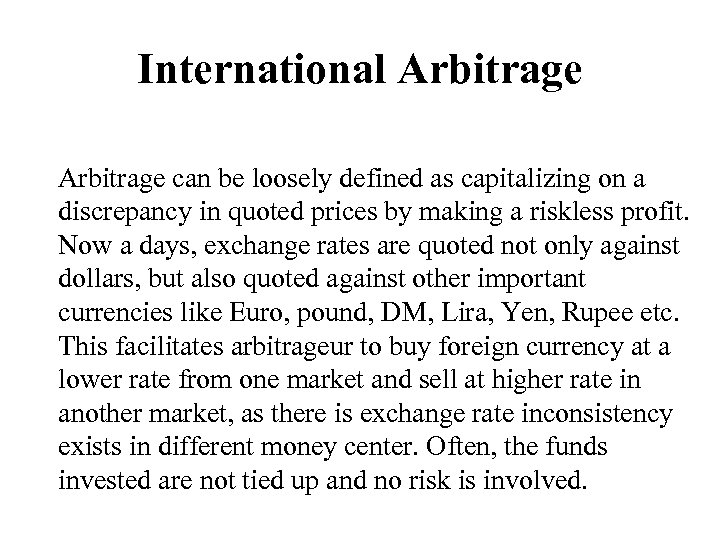International Arbitrage can be loosely defined as capitalizing on a discrepancy in quoted prices by making a riskless profit. Now a days, exchange rates are quoted not only against dollars, but also quoted against other important currencies like Euro, pound, DM, Lira, Yen, Rupee etc. This facilitates arbitrageur to buy foreign currency at a lower rate from one market and sell at higher rate in another market, as there is exchange rate inconsistency exists in different money center. Often, the funds invested are not tied up and no risk is involved.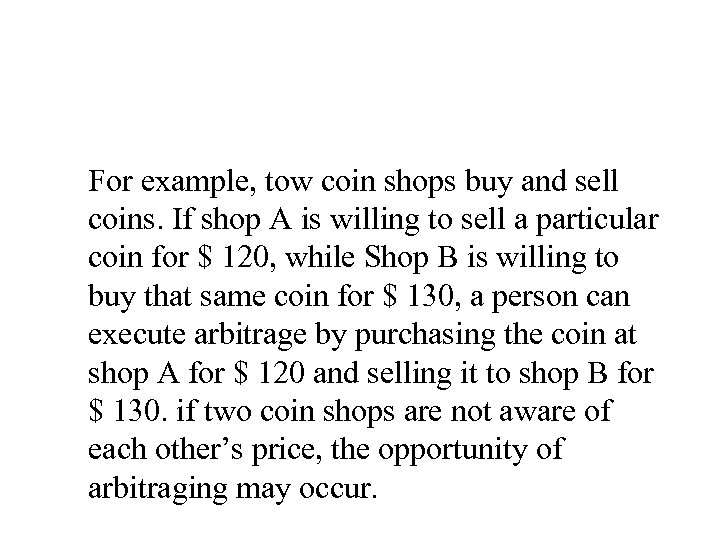For example, tow coin shops buy and sell coins. If shop A is willing to sell a particular coin for \$ 120, while Shop B is willing to buy that same coin for \$ 130, a person can execute arbitrage by purchasing the coin at shop A for \$ 120 and selling it to shop B for \$ 130. if two coin shops are not aware of each other’s price, the opportunity of arbitraging may occur.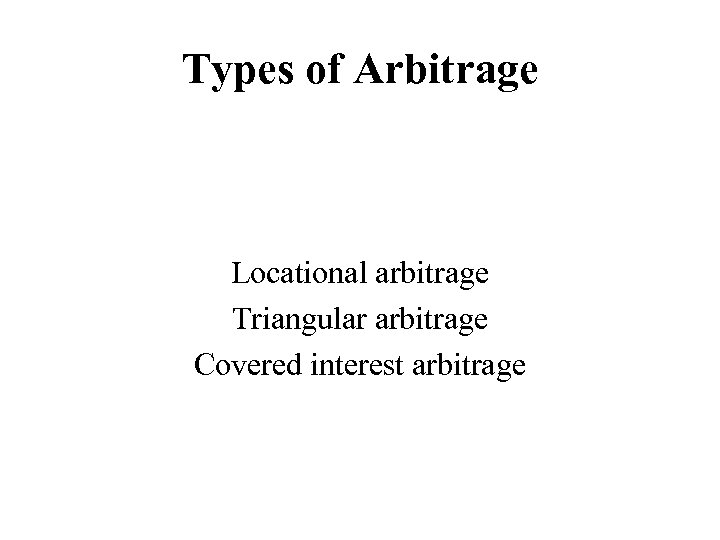Types of Arbitrage Locational arbitrage Triangular arbitrage Covered interest arbitrage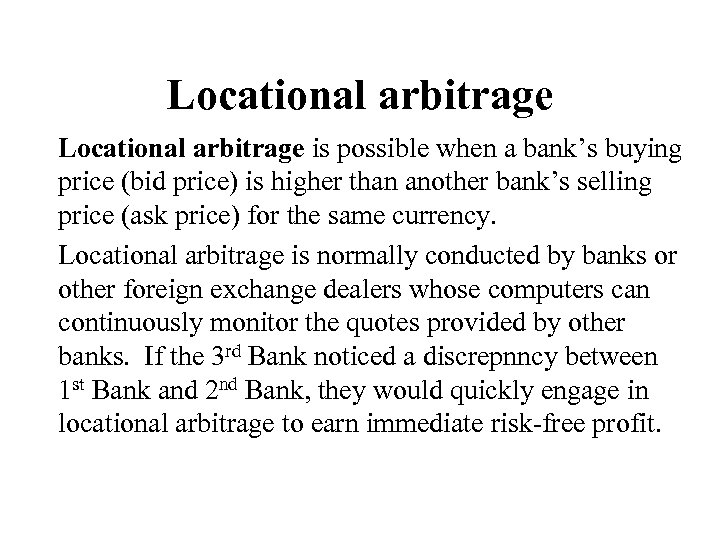Locational arbitrage is possible when a bank’s buying price (bid price) is higher than another bank’s selling price (ask price) for the same currency. Locational arbitrage is normally conducted by banks or other foreign exchange dealers whose computers can continuously monitor the quotes provided by other banks. If the 3 rd Bank noticed a discrepnncy between 1 st Bank and 2 nd Bank, they would quickly engage in locational arbitrage to earn immediate risk-free profit.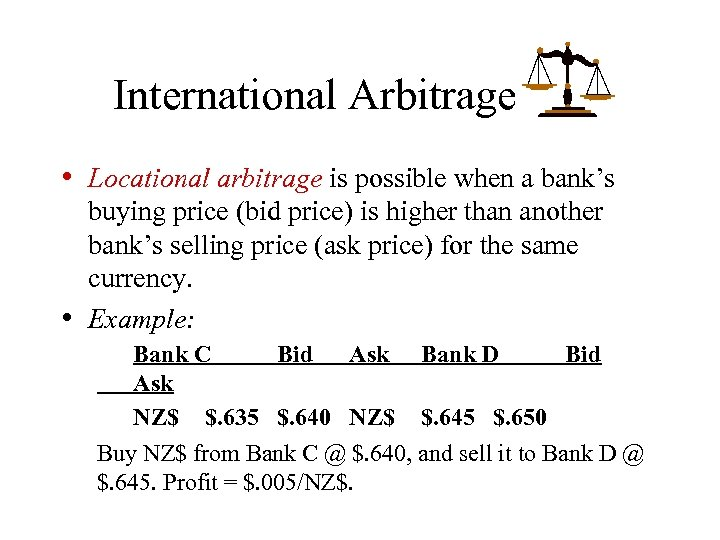International Arbitrage • Locational arbitrage is possible when a bank’s • buying price (bid price) is higher than another bank’s selling price (ask price) for the same currency. Example: Bank C Bid Ask Bank D Bid Ask NZ\$ \$. 635 \$. 640 NZ\$ \$. 645 \$. 650 Buy NZ\$ from Bank C @ \$. 640, and sell it to Bank D @ \$. 645. Profit = \$. 005/NZ\$.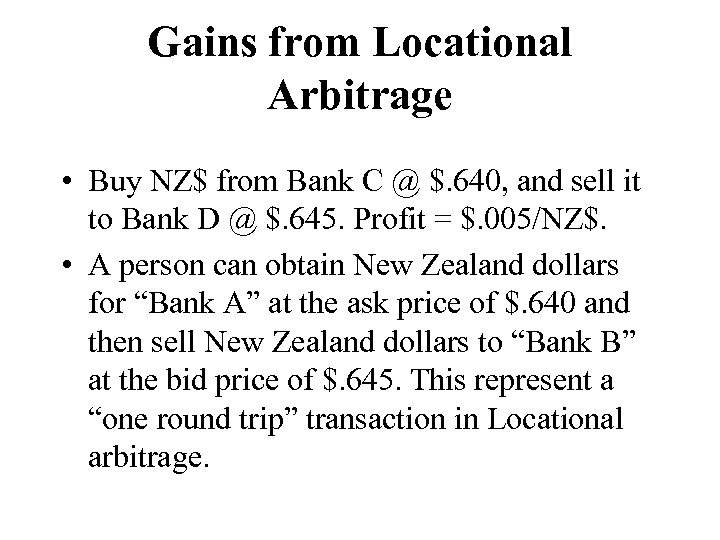Gains from Locational Arbitrage • Buy NZ\$ from Bank C @ \$. 640, and sell it to Bank D @ \$. 645. Profit = \$. 005/NZ\$. • A person can obtain New Zealand dollars for “Bank A” at the ask price of \$. 640 and then sell New Zealand dollars to “Bank B” at the bid price of \$. 645. This represent a “one round trip” transaction in Locational arbitrage.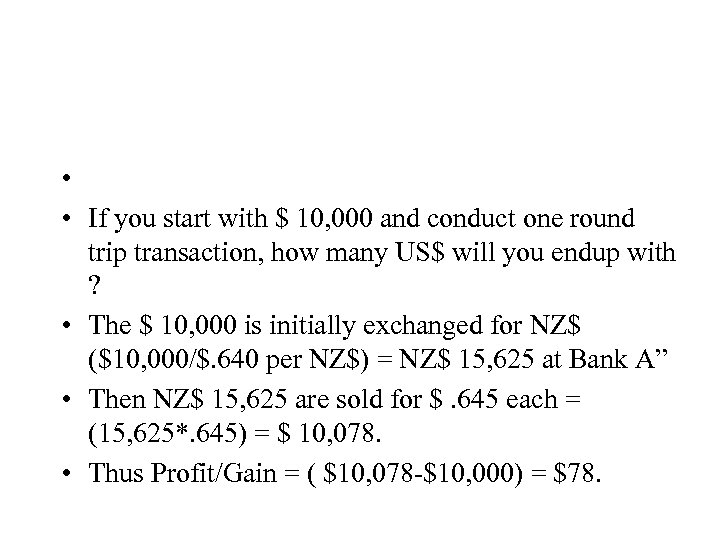• • If you start with \$ 10, 000 and conduct one round trip transaction, how many US\$ will you endup with ? • The \$ 10, 000 is initially exchanged for NZ\$ (\$10, 000/\$. 640 per NZ\$) = NZ\$ 15, 625 at Bank A” • Then NZ\$ 15, 625 are sold for \$. 645 each = (15, 625*. 645) = \$ 10, 078. • Thus Profit/Gain = ( \$10, 078 -\$10, 000) = \$78.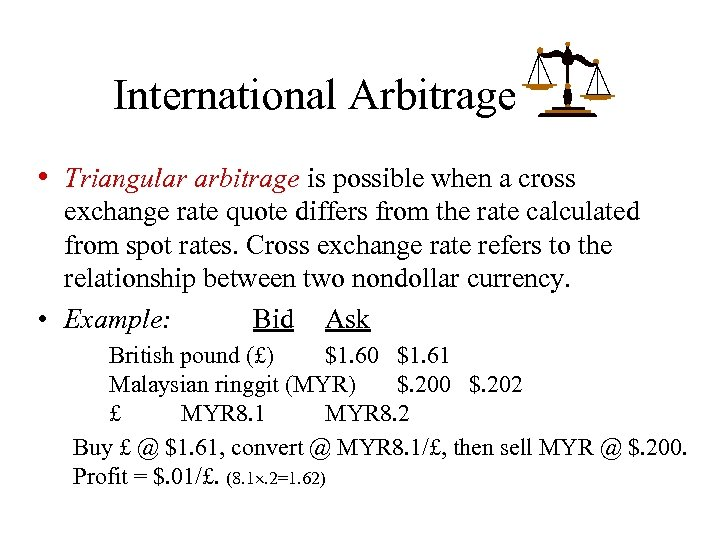International Arbitrage • Triangular arbitrage is possible when a cross exchange rate quote differs from the rate calculated from spot rates. Cross exchange rate refers to the relationship between two nondollar currency. • Example: Bid Ask British pound (£) \$1. 60 \$1. 61 Malaysian ringgit (MYR) \$. 200 \$. 202 £ MYR 8. 1 MYR 8. 2 Buy £ @ \$1. 61, convert @ MYR 8. 1/£, then sell MYR @ \$. 200. Profit = \$. 01/£. (8. 1. 2=1. 62)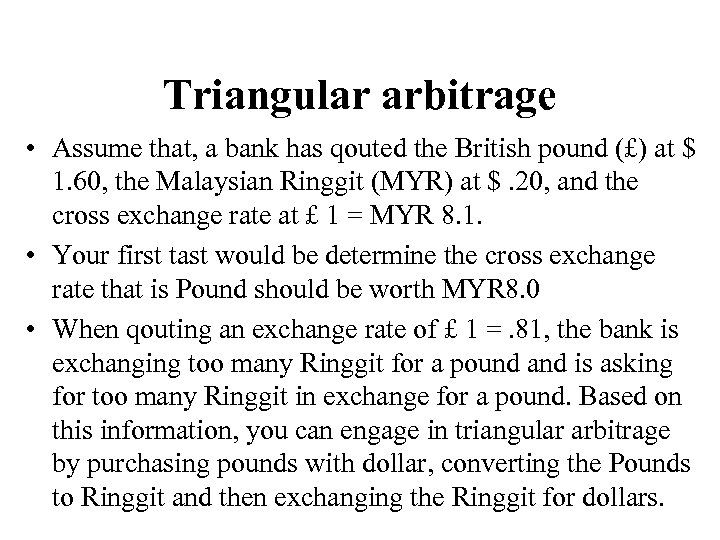Triangular arbitrage • Assume that, a bank has qouted the British pound (£) at \$ 1. 60, the Malaysian Ringgit (MYR) at \$. 20, and the cross exchange rate at £ 1 = MYR 8. 1. • Your first tast would be determine the cross exchange rate that is Pound should be worth MYR 8. 0 • When qouting an exchange rate of £ 1 =. 81, the bank is exchanging too many Ringgit for a pound and is asking for too many Ringgit in exchange for a pound. Based on this information, you can engage in triangular arbitrage by purchasing pounds with dollar, converting the Pounds to Ringgit and then exchanging the Ringgit for dollars.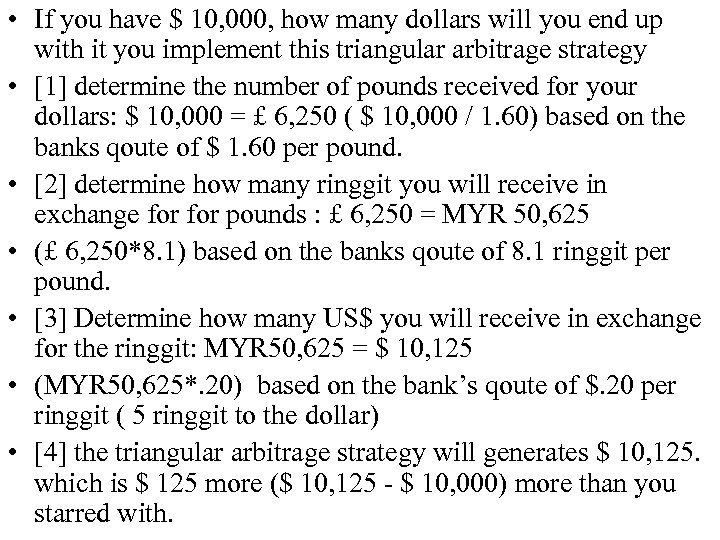• If you have \$ 10, 000, how many dollars will you end up with it you implement this triangular arbitrage strategy •  determine the number of pounds received for your dollars: \$ 10, 000 = £ 6, 250 ( \$ 10, 000 / 1. 60) based on the banks qoute of \$ 1. 60 per pound. •  determine how many ringgit you will receive in exchange for pounds : £ 6, 250 = MYR 50, 625 • (£ 6, 250*8. 1) based on the banks qoute of 8. 1 ringgit per pound. •  Determine how many US\$ you will receive in exchange for the ringgit: MYR 50, 625 = \$ 10, 125 • (MYR 50, 625*. 20) based on the bank’s qoute of \$. 20 per ringgit ( 5 ringgit to the dollar) •  the triangular arbitrage strategy will generates \$ 10, 125. which is \$ 125 more (\$ 10, 125 - \$ 10, 000) more than you starred with.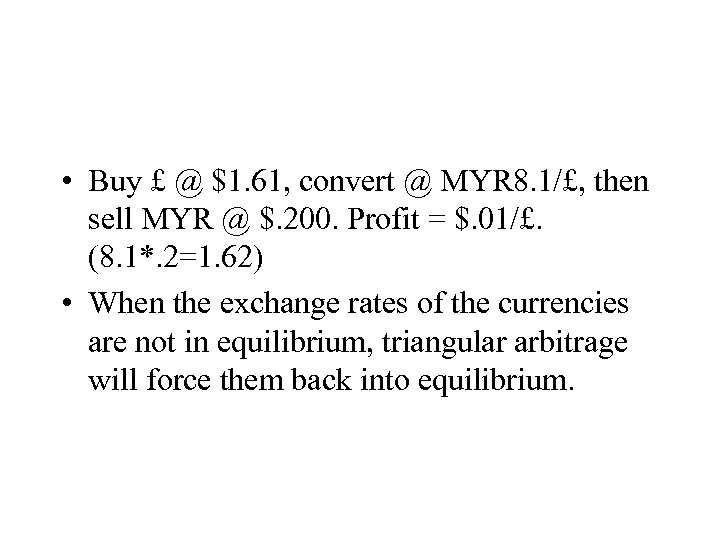• Buy £ @ \$1. 61, convert @ MYR 8. 1/£, then sell MYR @ \$. 200. Profit = \$. 01/£. (8. 1*. 2=1. 62) • When the exchange rates of the currencies are not in equilibrium, triangular arbitrage will force them back into equilibrium.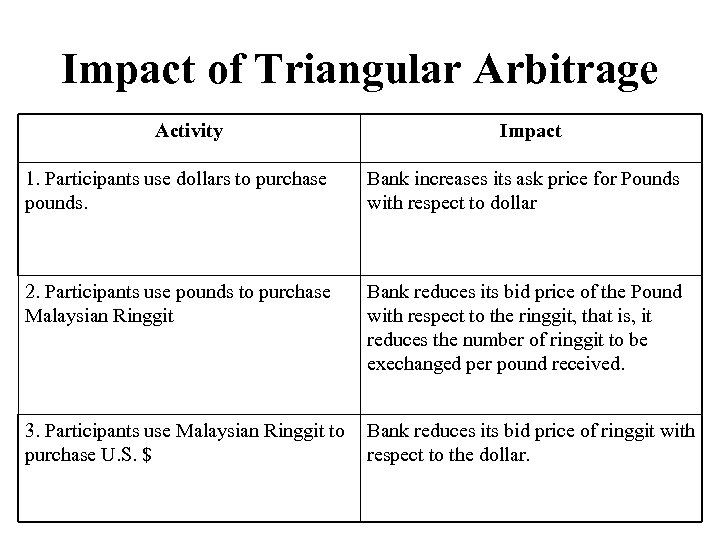Impact of Triangular Arbitrage Activity Impact 1. Participants use dollars to purchase pounds. Bank increases its ask price for Pounds with respect to dollar 2. Participants use pounds to purchase Malaysian Ringgit Bank reduces its bid price of the Pound with respect to the ringgit, that is, it reduces the number of ringgit to be exechanged per pound received. 3. Participants use Malaysian Ringgit to Bank reduces its bid price of ringgit with purchase U. S. \$ respect to the dollar.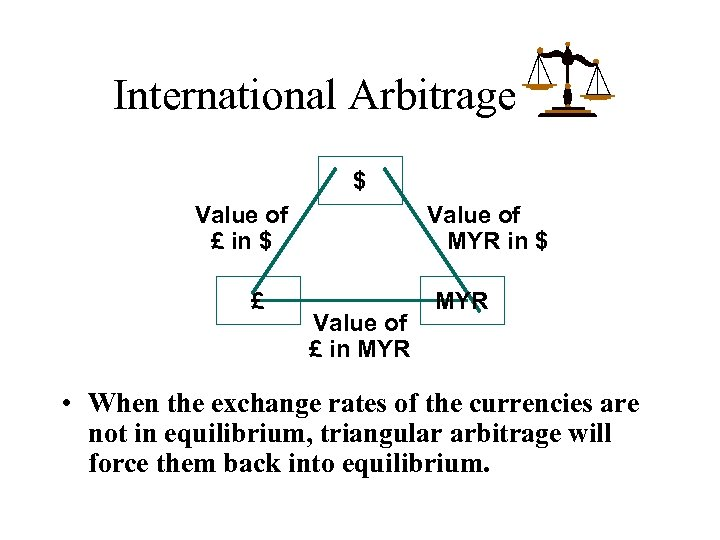International Arbitrage \$ Value of £ in \$ £ Value of MYR in \$ Value of £ in MYR • When the exchange rates of the currencies are not in equilibrium, triangular arbitrage will force them back into equilibrium.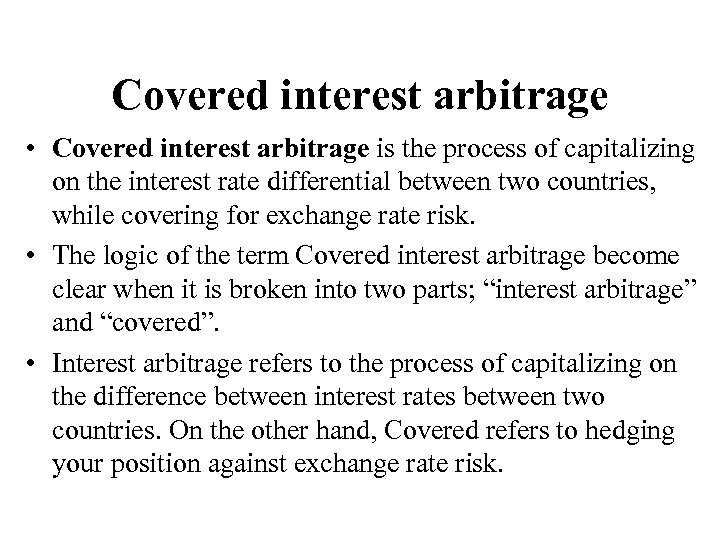Covered interest arbitrage • Covered interest arbitrage is the process of capitalizing on the interest rate differential between two countries, while covering for exchange rate risk. • The logic of the term Covered interest arbitrage become clear when it is broken into two parts; “interest arbitrage” and “covered”. • Interest arbitrage refers to the process of capitalizing on the difference between interest rates between two countries. On the other hand, Covered refers to hedging your position against exchange rate risk.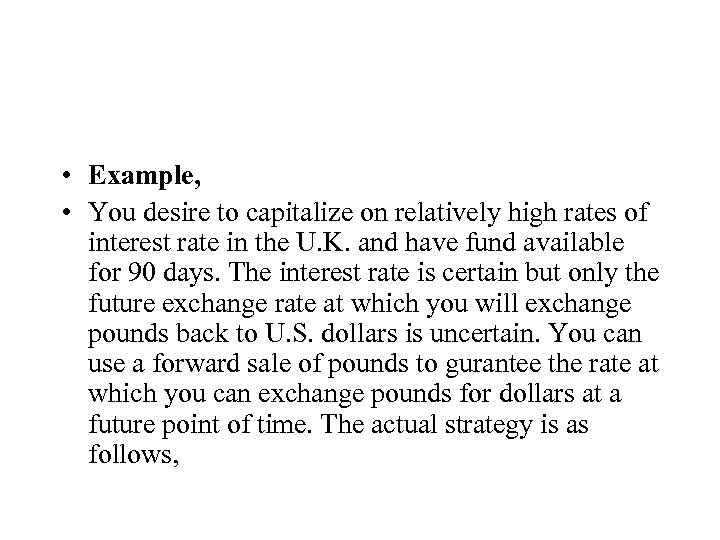• Example, • You desire to capitalize on relatively high rates of interest rate in the U. K. and have fund available for 90 days. The interest rate is certain but only the future exchange rate at which you will exchange pounds back to U. S. dollars is uncertain. You can use a forward sale of pounds to gurantee the rate at which you can exchange pounds for dollars at a future point of time. The actual strategy is as follows,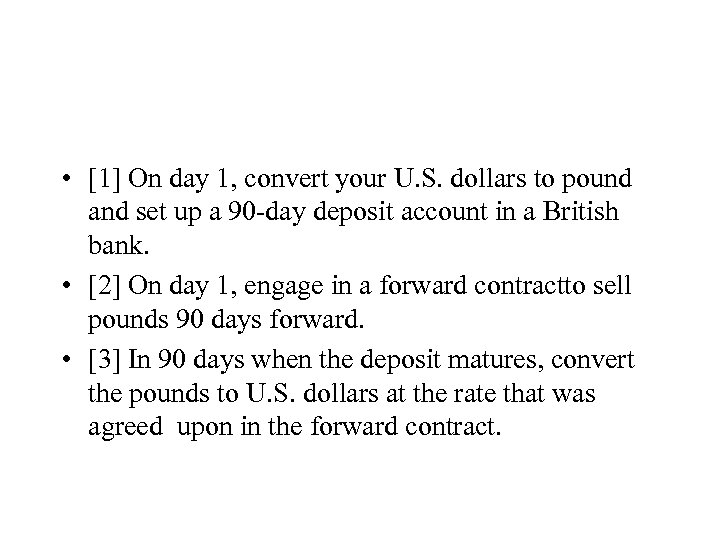•  On day 1, convert your U. S. dollars to pound and set up a 90 -day deposit account in a British bank. •  On day 1, engage in a forward contractto sell pounds 90 days forward. •  In 90 days when the deposit matures, convert the pounds to U. S. dollars at the rate that was agreed upon in the forward contract.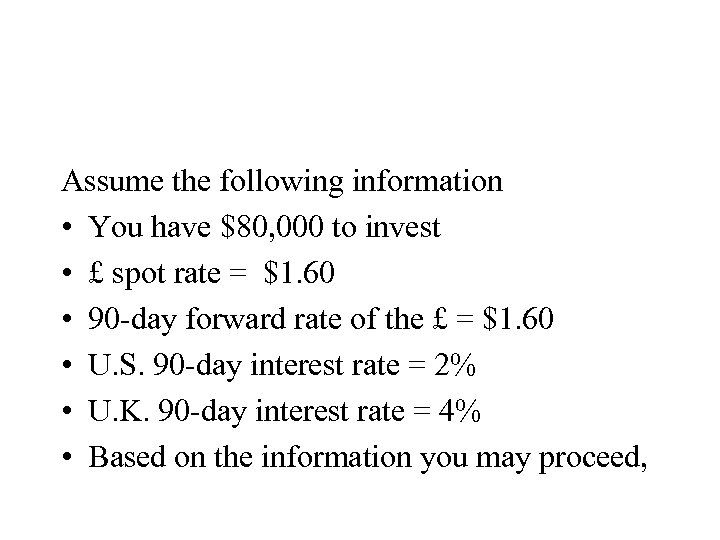Assume the following information • You have \$80, 000 to invest • £ spot rate = \$1. 60 • 90 -day forward rate of the £ = \$1. 60 • U. S. 90 -day interest rate = 2% • U. K. 90 -day interest rate = 4% • Based on the information you may proceed,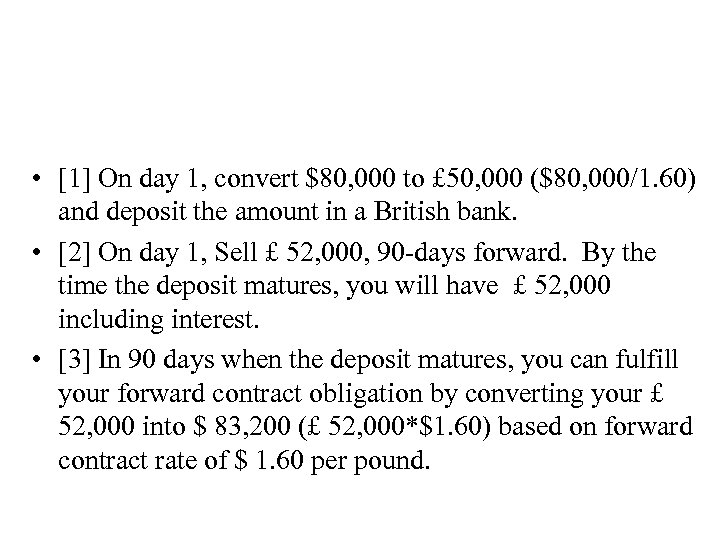•  On day 1, convert \$80, 000 to £ 50, 000 (\$80, 000/1. 60) and deposit the amount in a British bank. •  On day 1, Sell £ 52, 000, 90 -days forward. By the time the deposit matures, you will have £ 52, 000 including interest. •  In 90 days when the deposit matures, you can fulfill your forward contract obligation by converting your £ 52, 000 into \$ 83, 200 (£ 52, 000*\$1. 60) based on forward contract rate of \$ 1. 60 per pound.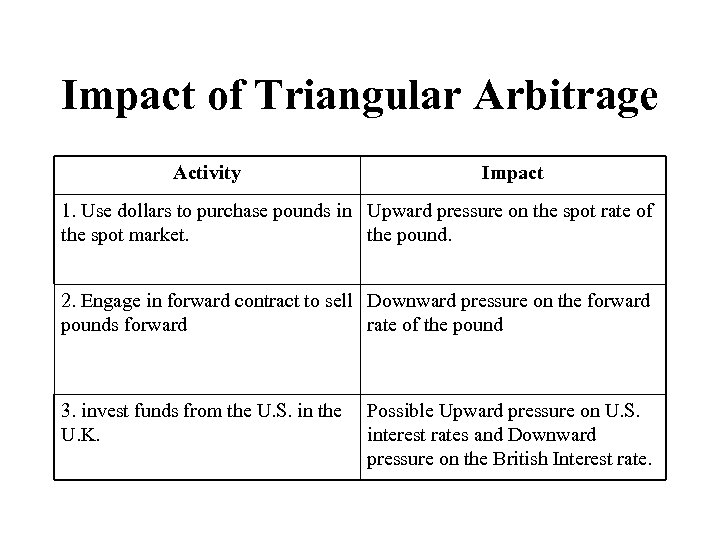Impact of Triangular Arbitrage Activity Impact 1. Use dollars to purchase pounds in Upward pressure on the spot rate of the spot market. the pound. 2. Engage in forward contract to sell Downward pressure on the forward pounds forward rate of the pound 3. invest funds from the U. S. in the U. K. Possible Upward pressure on U. S. interest rates and Downward pressure on the British Interest rate.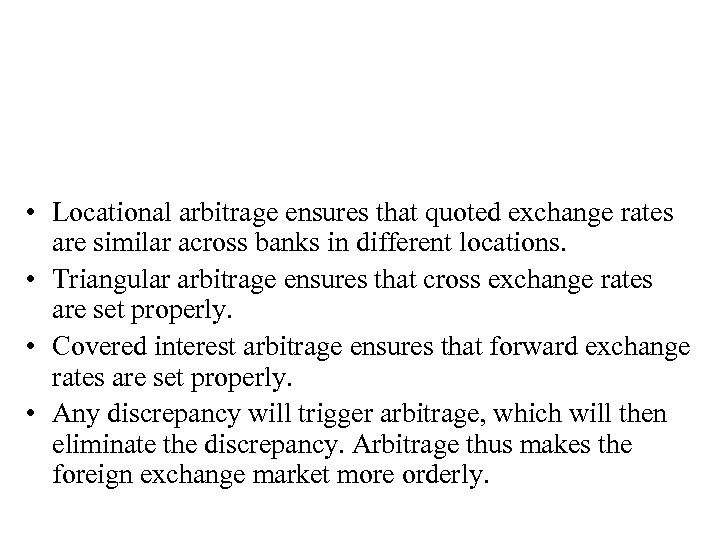• Locational arbitrage ensures that quoted exchange rates are similar across banks in different locations. • Triangular arbitrage ensures that cross exchange rates are set properly. • Covered interest arbitrage ensures that forward exchange rates are set properly. • Any discrepancy will trigger arbitrage, which will then eliminate the discrepancy. Arbitrage thus makes the foreign exchange market more orderly.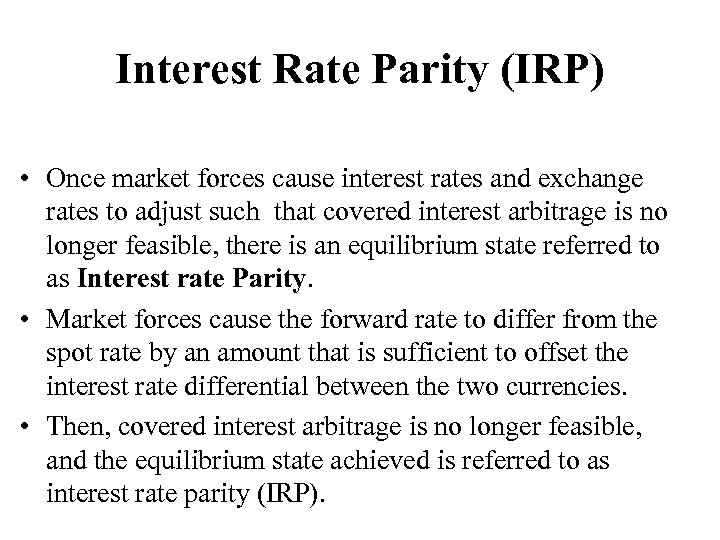Interest Rate Parity (IRP) • Once market forces cause interest rates and exchange rates to adjust such that covered interest arbitrage is no longer feasible, there is an equilibrium state referred to as Interest rate Parity. • Market forces cause the forward rate to differ from the spot rate by an amount that is sufficient to offset the interest rate differential between the two currencies. • Then, covered interest arbitrage is no longer feasible, and the equilibrium state achieved is referred to as interest rate parity (IRP).